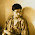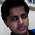### Number of Colour Changes

Source: A Quant Company Placement Test 2010 at IITB

Problem: You are given an urn with 100 balls (50 black and 50 white). You pick balls from urn one by one without replacements until all the balls are out. A black followed by a white or a white followed by a black is "a colour change". Calculate the expected number of colour changes if the balls are being picked randomly from the urn.

Update (Oct 30, 2010):

Update (Nov 05, 2010):
A different solution posted by Piyush Sao (5th year Dual Degree Elec Student, IIT Madras) in comments!

1.There are 99 possible pairs of balls.
The probability that one pair has color change is ((50/100)*(50/99)+(50/100)*(50/99)) which equals 50/99. There are 99 such pairs. So the average equals 50/99 * 99 =50.

2.Elegant solution. Thanks.

Explaining in detail so that everyone sees this is mathematically correct:

There are 99 positions. Let X_i be a random variable taking value 1 if i_th position has a colour change and zero otherwise.

We have to find expected value of E[X_1 + X_2 + ... + X_99]

Since all X_i are equivalent, the answer is 99*E[X_i]

E[X_i] = ((50/100)*(50/99)+(50/100)*(50/99)) = 50/99

:)

1.I am bit confused about equivalent of X_i's. Prob(X_i = 1) is different for each i, because (i-1) balls are drawn before ith position without replacement. Can you explain me in detail about equivalence of X_i?

2.I see how this may seem intuitive, but here's a proof for why this is the case (though I'd urge the reader to think from a more fundamental combinatorics perspective to see why this happens as well)

As seen in other answers, P(X1=1) = 50/99
P(X2=1) = P(X1=0, X2 = 1) + P(X1=1, X2=1) = P(X2=1|X1=0)*P(X1=0) + P(X2=1|X1=1)*P(X1=1) = (50/98)*(49/99) + (49/98)*(50/99) = 50/99 = P(X1= 1). So recursively, for sufficiently small n, this holds. This explicitly proves equivalence of X_i's.

3.This comment has been removed by the author.

4.I've a slightly different solution.

there are 100! ways it can be taken out. Now we count number of permutation( P_i) in which ith place will have a "colour change"
so it will be
I can place 50 ways a black ball at ith place and in 50 ways white ball at i+1th place rest 98 places can be arranged in 98! ways. similarlily white ball at ith place and black at i+1th place

So P_i = 50*50*2*(98!)

so total number of "colour change"
= summation(P_i;i=1 to 99)
=P_i*99=50*50*2*98!*99=50*100!

So expected number of color change = 50*100!/100! = 50

1.So, basically, it doesn't matter if the balls are taken with or without replacement 100 times? This is the part I am a little confused about!

5.@piyush.. very elegant.. thanks :)

6.This comment has been removed by a blog administrator.

7.99 pairs of balls, consider this as binary representation, 1 for change, 0 for no-change. so, we have to fing Expected number of 1's in 99 digit binary number. And as P[i] = P[99 - i] in that, we get expected number to be 50.

8.@Ameya (Stainless).. Why do you say that all binary representations are equally likely? In fact its not.
01 followed by 97 times 0 has probability zero as it would mean2 balls of one colour and 98 balls of another colour. Hence, I think your solution will not work. Am I missing something?

9.I actually checked whether all are equally likely or not.. and they are...
You can prove it easily...
HINT:
Try and exchange 1-0 by 0-1 at any change.. and see if the probability is same or not.
So, usign that you can prove that probability for each binary representation is same.

10.And ya.. you are right... I missed the case of 0 changes. which is no prob. P[i] = P[100-i] from i=1->99.

11.When you say, P[i]=P[99-i], what is P[i] here? and can you give exact proof?